Custom SearchOTHER PARAMETRIC EQUATIONS EXAMPLE. Find the equations of the tangent line and the normal line and the lengths of the tangent and the normal for the curve represented by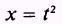and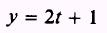atgiven thatand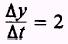SOLUTION.- Since t equals 1 we write x=1 and y=3 and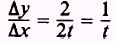so thatThe equation of the tangent line when t is equal to 1 is y-3=1(x-1) y=x+2 The equation of the normal line is y- 3 = -1(x- 1) y= -x+4 The length of the tangent isThe length of the normal is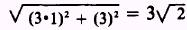EXAMPLE: Find the equations of the tangent line and the normal line and the lengths of the tangent and the normal to the curve represented by the parametric equationsand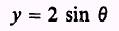at the point where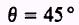given that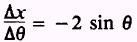and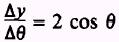SOLUTION. We know that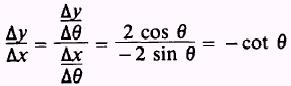Then at the point where, we have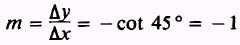If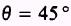is substituted in the parametric equations, thenand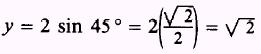The equation of the tangent line whenis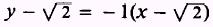orThe equation of the normal is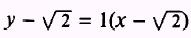or x-y=0 The length of the tangent isThe length of the normal is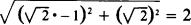The horizontal and vertical tangents of a curve can be found very easily when the curve is represented by parametric equations. The slope of a curve at any point equals zero when the tangent line is parallel to the X axis. In parametric equations, if x = x(t) and y = y(t), then the horizontal and vertical tangents can be found easily by setting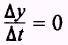and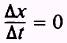For the horizontal tangent solve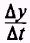equals zero for t and for the vertical tangent solve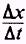equals zero for t. EXAMPLE: Find the points of contact of the horizontal and the vertical tangents to the curve represented by the parametric equationsand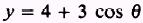Plot the graph of the curve by takingfrom 0 to 360 in increments of 30, given thatand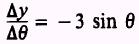Figure 3-7.-Ellipse. SOLUTION: The graph of the curve shows that the figure is an ellipse, figure 3-7; consequently, it will have two horizontal and two vertical tangents. The coordinates of the horizontal tangent points are found by first setting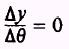This gives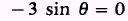so thatandSubstituting 0, we have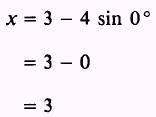and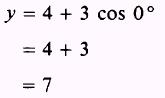Substituting 180, we obtain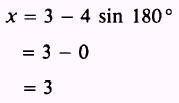and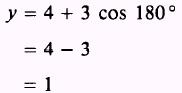The coordinates of the points of contact of the horizontal tangents to the ellipse are (3,1) and (3,7). The coordinates of the vertical tangent points of contact are found by settingWe findfrom which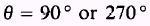Substituting 90, we obtain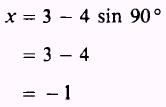and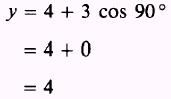Substituting 270 gives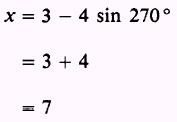and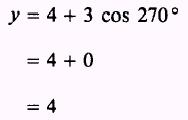The coordinates of the points of contact of the vertical tangents to the ellipse are (- 1,4) and (7,4).Integrated Publishing, Inc. - A (SDVOSB) Service Disabled Veteran Owned Small Business